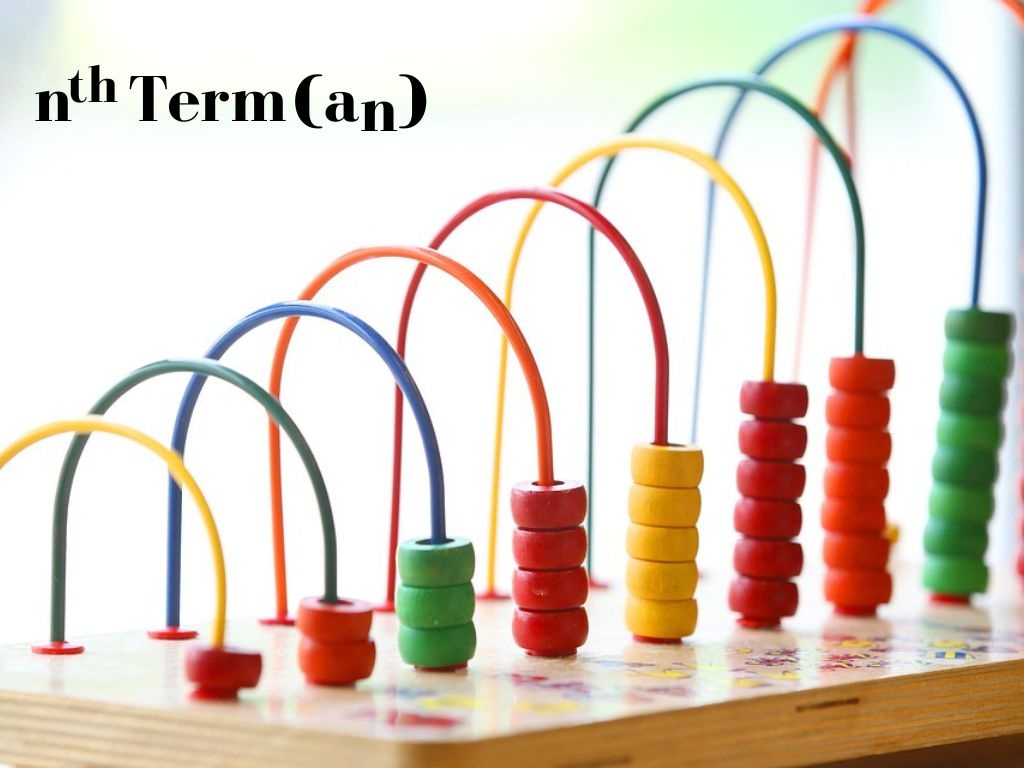## nth Term (General Term) of an Arithmetic Progression Class 10th

Derivation To find the nth term (general term) of an Arithmetic Progression, First of all, We know the general form of an Arithmetic Progression which is given as a, a + d, a + 2d, a + 3d, a + 4d,……………..a + (n – 1)d Here, we can see that the first term is a. …